# GED Math : Square Roots and Radicals

## Example Questions

← Previous 1 3 4

### Example Question #1 : Square Roots And Radicals

Simplify: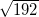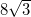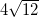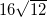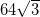Explanation: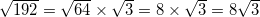An alternate solution is: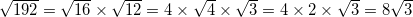### Example Question #2 : Square Roots And Radicals

Simplify: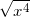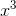Explanation: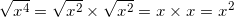### Example Question #3 : Square Roots And Radicals

Simplify: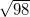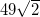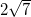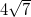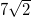Explanation: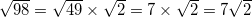### Example Question #4 : Square Roots And Radicals

Simplify: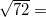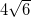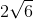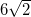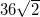Explanation: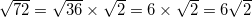### Example Question #5 : Square Roots And Radicals

Simplify: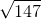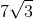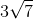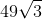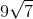Explanation: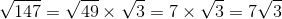### Example Question #6 : Square Roots And Radicals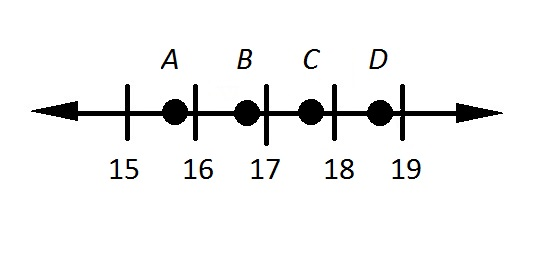Refer to the above number line. What point most likely represents the square root of 280?

Do not use a calculator.Explanation: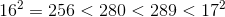Therefore,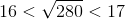making the correct choice.

### Example Question #7 : Square Roots And Radicals

Simplify: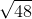Do not use a calculator.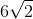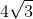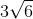The expression is in simplest form.Explanation:

The prime factorization of 48 is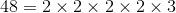.

Rewrite, and use the product of radicals property to simplify: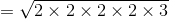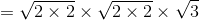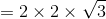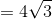### Example Question #7 : Square Roots And Radicals

Simplify: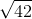Do not use a calculator.

The expression is in simplest form.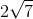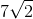The expression is in simplest form.

Explanation:

The prime factorization of 42 is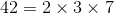.

Since 42 is the product of distinct primes, it has no perfect square factors, and, therefore, its square root cannot be simplified further. It is already in simplifed form.

### Example Question #9 : Square Roots And Radicals

Factor: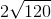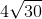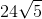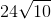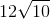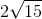Explanation:

In order to factor the radical, we will need to rewrite 120 as multiples of perfect squares.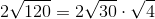Reduce the known term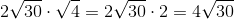The value of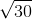cannot be factored any further.

The answer is:### Example Question #8 : Square Roots And Radicals

Factor: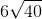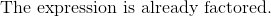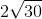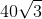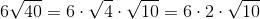The answer is: